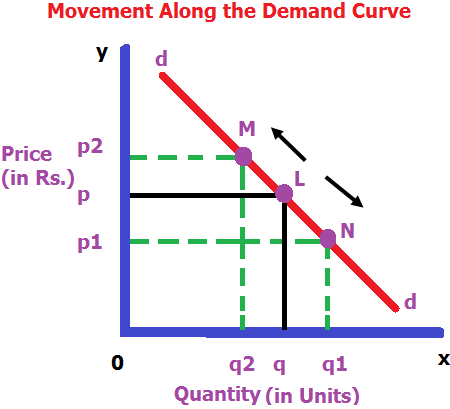# Concept of Movement along the Demand

## (a) MEANING OF MOVEMENT ALONG THE DEMAND CURVE

Keeping all other factors the same, when there is a change in demand of a commodity due to change in price, it is referred to as the change in quantity demanded. It is shown as a movement along the demand curve when expressed graphically.

## (b) UPWARD MOVEMENT OF DEMAND CURVE

• When the price of the commodity rises, the quantity demanded falls. It leads to the upward movement of the demand curve.
• It is also known as contraction of demand.

## (c) DOWNWARD MOVEMENT OF DEMAND CURVE

• When the price of the commodity falls, the quantity demanded rises. It leads to the downward movement of the demand curve.
• It is also known as expansion of demand.

## (d) DIAGRAM## (e)EXPLANATION OF DIAGRAM

• When price rises from OP to OP2 demand falls from OQ to OQ2. This is known as contraction of demand.
• When price falls from OP to OP1 demand rises from OQ to OQ1. This is known as expansion of demand.

### Lets Practice

1. Contraction in demand leads to_________________ ?

(a) Upward movement along the demand curve

(b) Downward movement along the demand curve

(c) Leftward shift in demand curve

(d) Rightward shift in demand curve

(a) Upward movement along the demand curve

2. The change in demand of a commodity due to increase in its price is called:

(a) Extension in demand

(b) Contraction in demand

(c) Increase in demand

(d) Decrease in demand

(b) Contraction in demand

3.The upward movement along a demand curve is known as

(a) Contraction in demand

(b) Expansion in demand

(c) Either (a) or (b)

(d) None of the above